• rc的低通/高通/带通/带阻 滤波器设计，及传递函数计算
• 带通滤波器所使用的归一化变换是将s＋1／s代入低通传递函数公式得到高通滤波器的传递函数，如果将这一关系代入高通滤波器则获得一个带阻滤波器。图1表示了高通滤波器频率响应与变换后的带阻滤波器频率响应之间的等效...
• 3、带通：是指能通过某一频率范围内的频率分量、但将其他范围的频率分量衰减到极低水平的滤波器，与带阻滤波器的概念相对。一个模拟带通滤波器的例子是电阻-电感-电容电路(RLC circuit)。这些滤波器也可以用低通...
展开全部1、低通：(Low-pass filter)是容许低于截止频率的信32313133353236313431303231363533e4b893e5b19e31333366303732号通过，但高于截止频率的信号不能通过的电子滤波装置。2、高通：是一种让某一频率以上的信号分量通过，而对该频率以下的信号分量大大抑制的电容、电感与电阻等器件的组合装置。其特性在时域及频域中可分别用冲激响应及频率响应描述。3、带通：是指能通过某一频率范围内的频率分量、但将其他范围的频率分量衰减到极低水平的滤波器，与带阻滤波器的概念相对。一个模拟带通滤波器的例子是电阻-电感-电容电路(RLC circuit)。这些滤波器也可以用低通滤波器同高通滤波器组合来产生。4、带阻滤波器：是指能通过大多数频率分量、但将某些范围的频率分量衰减到极低水平的滤波器，与带通滤波器的概念相对。其中点阻滤波器(notch filter)是一种特殊的带阻滤波器，它的阻带范围极小，有着很高的Q值(Q Factor)。将输入电压同时作用于低通滤波器和高通滤波器，再将两个电路的输出电压求和，就可以得到带阻滤波器，如下图所示。其中低通滤波器的截止频率 应小于高通滤波器的截止频率 ，因此，电路的阻带为( - )。扩展资料低通原理利用：1、巴特沃斯滤波器巴特沃斯滤波器是滤波器的一种设计分类，其采用的是巴特沃斯传递函数，有高通、低通、带通、带阻等多种滤波器类型。巴特沃斯滤波器在通频带内外都有平稳的幅频特性，但有较长的过渡带，在过渡带上很容易造成失真。2、切比雪夫滤波器切比雪夫滤波器是滤波器的一种设计分类，其采用的是切比雪夫传递函数，也有高通、低通、带通、高阻、带阻等多种滤波器类型。同巴特沃斯滤波器相比，切比雪夫滤波器的过渡带很窄，但内部的幅频特性却很不稳定。高通种类：1、按照所采用的器件不同分类有源高通滤波器、无源高通滤波器。无源高通滤波器： 仅由无源元件(R、L 和C)组成的滤波器，它是利用电容和电感元件的电抗随频率的变化而变化的原理构成的。这类滤波器的优点是：电路比较简单，不需要直流电源供电，可靠性高；缺点是：通带内的信号有能量损耗，负载效应比较明显，使用电感元件时容易引起电磁感应，当电感L较大时滤波器的体积和重量都比较大，在低频域不适用。有源高通滤波器：由无源元件(一般用R和C)和有源器件(如集成运算放大器)组成。这类滤波器的优点是：通带内的信号不仅没有能量损耗，而且还可以放大，负载效应不明显，多级相联时相互影响很小。利用级联的简单方法很容易构成高阶滤波器，并且滤波器的体积小、重量轻、不需要磁屏蔽(由于不使用电感元件)；缺点是：通带范围受有源器件(如集成运算放大器)的带宽限制，需要直流电源供电，可靠性不如无源滤波器高，在高压、高频、大功率的场合不适用。2、按照滤波器的数学特性分为一阶高通滤波器、二阶高通滤波器等。
展开全文• 本文主要绘制常用滤波器的透视图，包括低通，带阻和带通滤波器。而这三类滤波器又各自包含了理想、巴特沃兹和高斯滤波器。如，低通滤波器就可以分为理想低通滤波器、n阶巴特沃兹滤波器和高斯低通滤波器。 第一：...

原文地址：http://blog.csdn.net/ljp1919/article/details/44781215

本文主要绘制常用滤波器的透视图，包括低通，带阻和带通滤波器。而这三类滤波器又各自包含了理想、巴特沃兹和高斯滤波器。如，低通滤波器就可以分为理想低通滤波器、n阶巴特沃兹滤波器和高斯低通滤波器。

第一：低通滤波器

1）理想低通滤波器

传递函数：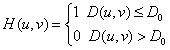透视图结果：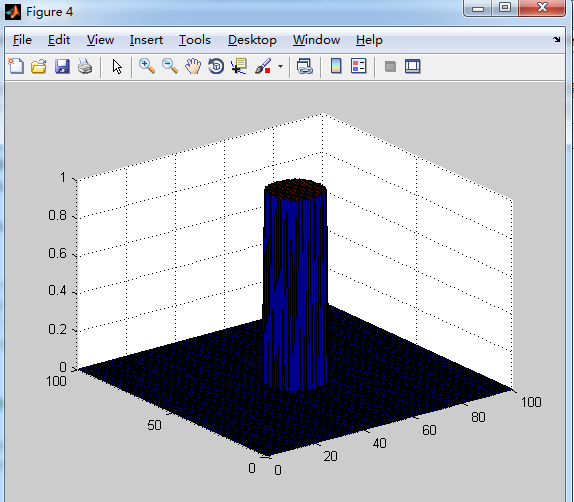代码：

[cpp] view
plain copya=100;
b=100;
U=0:a;
V=0:b;
M=length(U);N=length(V);
D0=10;%W=200;%D0是频带的中心半径;W是频带的宽度
x1=50;y1=50;
x0=-50;y0=-50;
m=fix(M/2); n=fix(N/2);
H=zeros(M,N);
n=8;
for u=1:M
for v=1:N
a=sqrt((U(u) - 50) .* (U(u)-50) + (V(v) - 50) .* (V(v) - 50));%D(u,v)的值
if(a<=D0)%理想陷波器
H(u,v)=1;
else
H(u,v)=0;
end
end
end
%在绘制高斯曲面的时候，加上下述代码，显示得美观
figure;
surf(U,V,H)

2）n阶巴特沃兹低通滤波器

传递函数：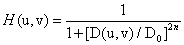透视图结果：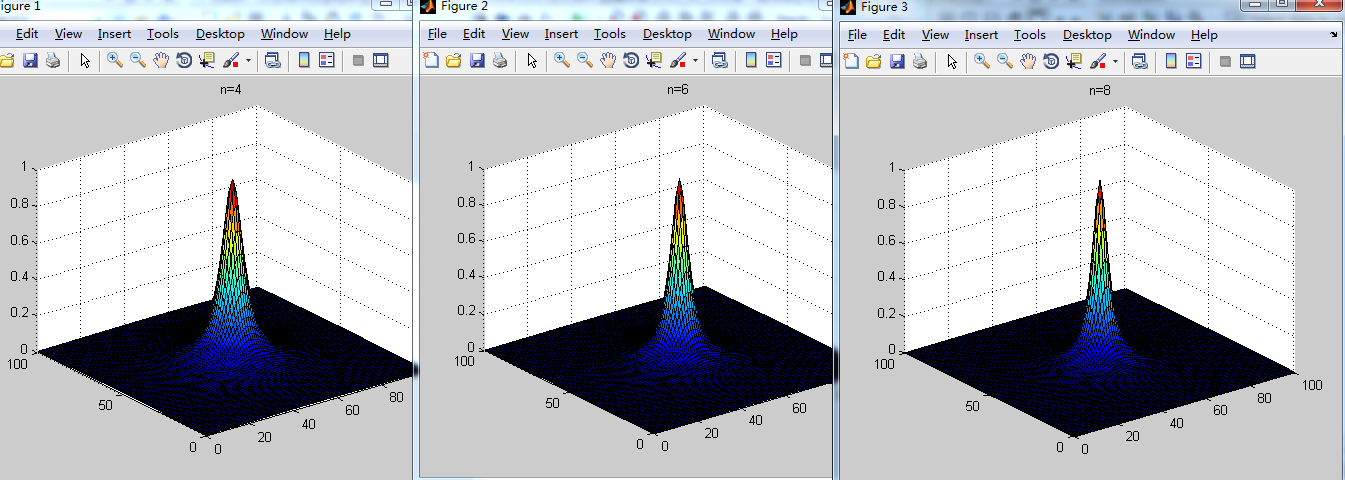代码：

[cpp] view
plain copya=100;
b=100;
U=0:a;
V=0:b;
M=length(U);N=length(V);
D0=10;%W=200;%D0是频带的中心半径;W是频带的宽度
x1=50;y1=50;
x0=-50;y0=-50;
m=fix(M/2); n=fix(N/2);
H=zeros(M,N);
n=8;
for u=1:M
for v=1:N
a=sqrt((U(u) - 50) .* (U(u)-50) + (V(v) - 50) .* (V(v) - 50));%D(u,v)的值
b=1+(a/D0)^2*n;
H(u,v)=1/b;%尝试下，非理想滤波器，如巴特沃兹或者高斯
end
end
figure;
surf(U,V,H)
title('n=8')

3）高斯低通滤波器

传递函数：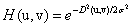透视图结果：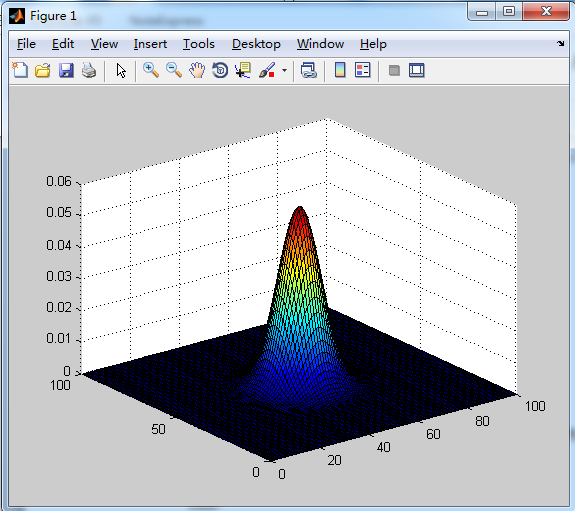代码：

[cpp] view
plain copya=100;
b=100;
U=0:a;
V=0:b;
M=length(U);N=length(V);
D0=10; %D0是频带的中心半径;W是频带的宽度
x1=50;y1=50;
x0=-50;y0=-50;
m=fix(M/2); n=fix(N/2);
H=zeros(M,N);

for u=1:M
for v=1:N
D1=((u-m-x0)^2+(v-n-y0).^2)^0.5;
D2=((u-m+x0)^2+(v-n+y0).^2)^0.5;
D11=((u-m-x1)^2+(v-n-y1).^2)^0.5;
D21=((u-m+x1)^2+(v-n+y1).^2)^0.5;
%高斯低通曲面
H(u,v) = (U(u) - 50) .* (U(u)-50) + (V(v) - 50) .* (V(v) - 50);

end
end
%在绘制高斯曲面的时候，加上下述代码，显示得美观
fangcha=50;
H = -H/(2*fangcha);
H = exp(H) / (sqrt(2*pi) * sqrt(fangcha));

surf(U,V,H)

第二：带阻滤波器

1）理想带阻滤波器

传递函数：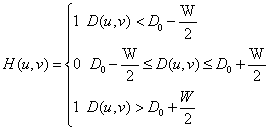透视图结果：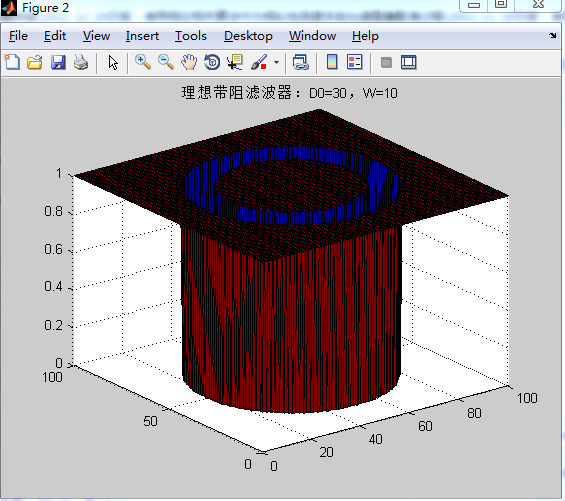代码：

[cpp] view
plain copy% %绘制函数剖面线
% [u,v] = meshgrid(-400:400, -400:400);
a=100;%图像的尺寸，长
b=100;%图像的尺寸，宽
U=0:a;
V=0:b;
M=length(U);N=length(V);
D0=30;%W=200;%D0是频带的中心半径;W是频带的宽度
x1=50;y1=50;
x0=-50;y0=-50;
m=fix(M/2);n=fix(N/2);
H=zeros(M,N);
% n=8;
W=10;%W是频带的宽度
for u=1:M
for v=1:N
%         D1=((u-m-x0)^2+(v-n-y0).^2)^0.5;
%         D2=((u-m+x0)^2+(v-n+y0).^2)^0.5;
%         D11=((u-m-x1)^2+(v-n-y1).^2)^0.5;
%         D21=((u-m+x1)^2+(v-n+y1).^2)^0.5;

%         % %设计带阻滤波器
%         H(u,v)=1-exp(-0.5*((D(u,v)^2-D0^2)/(D(u,v)*W))^2);
D=sqrt((U(u) - m) .* (U(u)-m) + (V(v) - n) .* (V(v) - n));%D(u,v)的值
if((D<D0-W/2) || (D>D0+W/2))%理想带阻滤波器
H(u,v)=1;
else
H(u,v)=0;
end

%         H(u,v)=1-exp(-0.5*((D1*D2/D0^2)));%尝试下，非理想滤波器，如巴特沃兹或者高斯
%       a=sqrt((U(u) - 50) .* (U(u)-50) + (V(v) - 50) .* (V(v) - 50));%D(u,v)的值
%       b=1+(a/D0)^2*n;
%       H(u,v)=1/b;%尝试下，非理想滤波器，如巴特沃兹或者高斯
end
end
%在绘制高斯曲面的时候，加上下述代码，显示得美观
% fangcha=50;
% H = -H/(2*fangcha);
% H = exp(H) / (sqrt(2*pi) * sqrt(fangcha));
figure;
surf(U,V,H)
title('理想带阻滤波器：D0=30，W=10')
% [X,Y] = meshgrid(-2:.2:2, -2:.2:5);
% Z = X .* exp(-X.^2 - Y.^2);
% surf(X,Y,Z)

2）n阶巴特沃兹带阻滤波器

传递函数：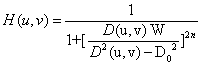透视图结果：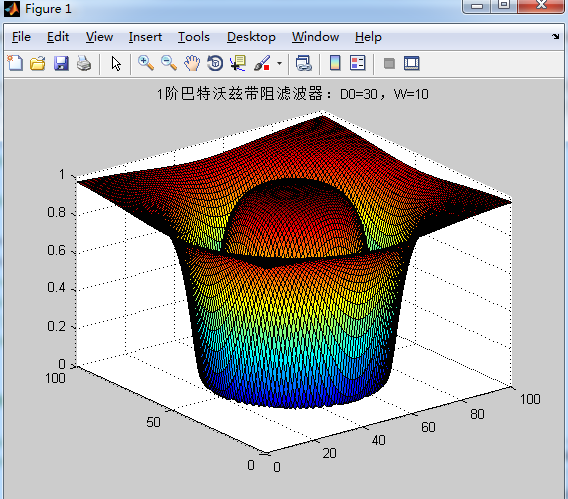代码：

[cpp] view
plain copy% %绘制函数剖面线
% [u,v] = meshgrid(-400:400, -400:400);
a=100;%图像的尺寸，长
b=100;%图像的尺寸，宽
U=0:a;
V=0:b;
M=length(U);N=length(V);
D0=30;%W=200;%D0是频带的中心半径;W是频带的宽度
x1=50;y1=50;
x0=-50;y0=-50;
m=fix(M/2);n=fix(N/2);
H=zeros(M,N);
n1=1;%巴特沃兹的阶数
W=10;%W是频带的宽度
for u=1:M
for v=1:N
%         D1=((u-m-x0)^2+(v-n-y0).^2)^0.5;
%         D2=((u-m+x0)^2+(v-n+y0).^2)^0.5;
%         D11=((u-m-x1)^2+(v-n-y1).^2)^0.5;
%         D21=((u-m+x1)^2+(v-n+y1).^2)^0.5;

%         % %设计带阻滤波器
%         H(u,v)=1-exp(-0.5*((D(u,v)^2-D0^2)/(D(u,v)*W))^2);
D=sqrt((U(u) - m) .* (U(u)-m) + (V(v) - n) .* (V(v) - n));%D(u,v)的值
%             if((D<D0-W/2) || (D>D0+W/2))%理想带阻滤波器
%                 H(u,v)=1;
%             else
%                 H(u,v)=0;
%             end
temp=1+(D*W/(D^2-D0^2))^2*n1;
H(u,v)=1/temp;
%         H(u,v)=1-exp(-0.5*((D1*D2/D0^2)));%尝试下，非理想滤波器，如巴特沃兹或者高斯
%       a=sqrt((U(u) - 50) .* (U(u)-50) + (V(v) - 50) .* (V(v) - 50));%D(u,v)的值
%       b=1+(a/D0)^2*n;
%       H(u,v)=1/b;%尝试下，非理想滤波器，如巴特沃兹或者高斯
end
end
%在绘制高斯曲面的时候，加上下述代码，显示得美观
% fangcha=50;
% H = -H/(2*fangcha);
% H = exp(H) / (sqrt(2*pi) * sqrt(fangcha));
figure;
surf(U,V,H)
title('1阶巴特沃兹带阻滤波器：D0=30，W=10')
% [X,Y] = meshgrid(-2:.2:2, -2:.2:5);
% Z = X .* exp(-X.^2 - Y.^2);
% surf(X,Y,Z)

3）高斯带阻滤波器

传递函数：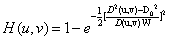透视图：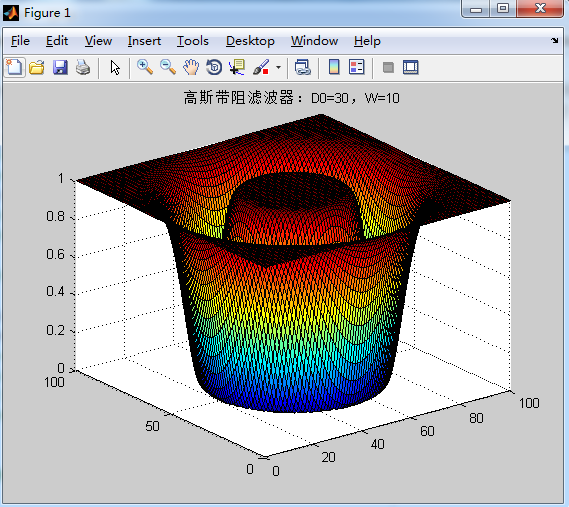代码：

[cpp] view
plain copy% %绘制函数剖面线
% [u,v] = meshgrid(-400:400, -400:400);
a=100;%图像的尺寸，长
b=100;%图像的尺寸，宽
U=0:a;
V=0:b;
M=length(U);N=length(V);
D0=30;%W=200;%D0是频带的中心半径;W是频带的宽度
x1=10;y1=10;
u0=-20;v0=20;%（u0,v0）的值对应移动中心
m=fix(M/2);n=fix(N/2);
H=zeros(M,N);
n1=2;%巴特沃兹的阶数
W=10;%W是频带的宽度
for u=1:M
for v=1:N
%         D1=((u-m-u0)^2+(v-n-v0).^2)^0.5;%（）
%         D2=((u-m+u0)^2+(v-n+v0).^2)^0.5;

%          D1=((U(u)-m-u0)^2+(V(v)-n-v0)^2)^0.5;%（）
%         D2=((U(u)-m+u0)^2+(V(v)-n+v0)^2)^0.5;

%         D11=((u-m-x1)^2+(v-n-y1).^2)^0.5;
%         D21=((u-m+x1)^2+(v-n+y1).^2)^0.5;

%         % %设计带阻滤波器
%         H(u,v)=1-exp(-0.5*((D(u,v)^2-D0^2)/(D(u,v)*W))^2);
%             D=sqrt((U(u) - m) .* (U(u)-m) + (V(v) - n) .* (V(v) - n));
D=sqrt((U(u) - m) .* (U(u)-m) + (V(v) - n) .* (V(v) - n));%D(u,v)的值
%             if((D<D0-W/2) || (D>D0+W/2))%理想带阻滤波器
%                 H(u,v)=1;
%             else
%                 H(u,v)=0;
%             end
temp=-0.5*((D^2-D0^2)/(D*W))^2;
H(u,v)=1-exp(temp);
%         H(u,v)=1-exp(-0.5*((D1*D2/D0^2)));%尝试下，非理想滤波器，如巴特沃兹或者高斯
%       a=sqrt((U(u) - 50) .* (U(u)-50) + (V(v) - 50) .* (V(v) - 50));%D(u,v)的值
%       b=1+(a/D0)^2*n;
%       H(u,v)=1/b;%尝试下，非理想滤波器，如巴特沃兹或者高斯
end
end
%在绘制高斯曲面的时候，加上下述代码，显示得美观
% fangcha=50;
% H = -H/(2*fangcha);
% H = exp(H) / (sqrt(2*pi) * sqrt(fangcha));
figure;
surf(U,V,H)
title('高斯带阻滤波器：D0=30，W=10')

第三：陷波器

1）理想陷波器

传递函数：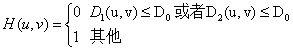透视图：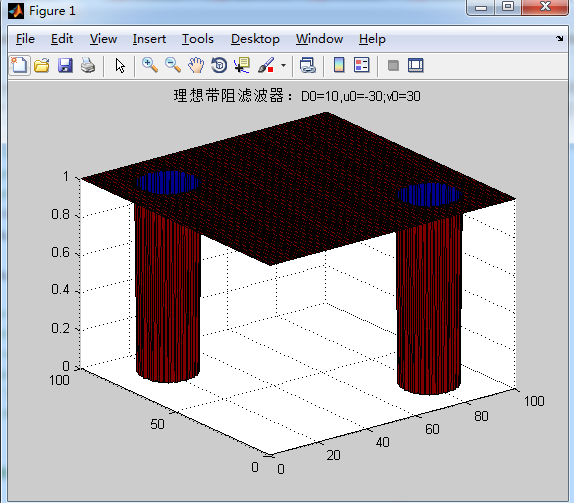代码：

[cpp] view
plain copy% %绘制函数剖面线
% [u,v] = meshgrid(-400:400, -400:400);
a=100;%图像的尺寸，长
b=100;%图像的尺寸，宽
U=0:a;
V=0:b;
M=length(U);N=length(V);
D0=10;%W=200;%D0是频带的中心半径;W是频带的宽度
x1=10;y1=10;
u0=-30;v0=30;%（u0,v0）的值对应移动中心
m=fix(M/2);n=fix(N/2);
H=zeros(M,N);
n1=2;%巴特沃兹的阶数
W=10;%W是频带的宽度
for u=1:M
for v=1:N
%         D1=((u-m-u0)^2+(v-n-v0).^2)^0.5;%（）
%         D2=((u-m+u0)^2+(v-n+v0).^2)^0.5;

D1=((U(u)-m-u0)^2+(V(v)-n-v0)^2)^0.5;%（）
D2=((U(u)-m+u0)^2+(V(v)-n+v0)^2)^0.5;

%         D11=((u-m-x1)^2+(v-n-y1).^2)^0.5;
%         D21=((u-m+x1)^2+(v-n+y1).^2)^0.5;

%         % %设计带阻滤波器
%         H(u,v)=1-exp(-0.5*((D(u,v)^2-D0^2)/(D(u,v)*W))^2);
%             D=sqrt((U(u) - m) .* (U(u)-m) + (V(v) - n) .* (V(v) - n));
%             D=sqrt((U(u) - m) .* (U(u)-m) + (V(v) - n) .* (V(v) - n));%D(u,v)的值
if((D1<=D0) || (D2<=D0))%理想带阻滤波器
H(u,v)=0;
else
H(u,v)=1;
end
%             temp=-0.5*((D^2-D0^2)/(D*W))^2;
%             H(u,v)=1-exp(temp);
%         H(u,v)=1-exp(-0.5*((D1*D2/D0^2)));%尝试下，非理想滤波器，如巴特沃兹或者高斯
%       a=sqrt((U(u) - 50) .* (U(u)-50) + (V(v) - 50) .* (V(v) - 50));%D(u,v)的值
%       b=1+(a/D0)^2*n;
%       H(u,v)=1/b;%尝试下，非理想滤波器，如巴特沃兹或者高斯
end
end
%在绘制高斯曲面的时候，加上下述代码，显示得美观
% fangcha=50;
% H = -H/(2*fangcha);
% H = exp(H) / (sqrt(2*pi) * sqrt(fangcha));
figure;
surf(U,V,H)
title('理想带阻滤波器：D0=10,u0=-30;v0=30')
% [X,Y] = meshgrid(-2:.2:2, -2:.2:5);
% Z = X .* exp(-X.^2 - Y.^2);
% surf(X,Y,Z)

2）n阶巴特沃兹陷波器：

传递函数：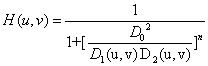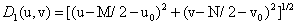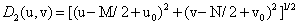透视图：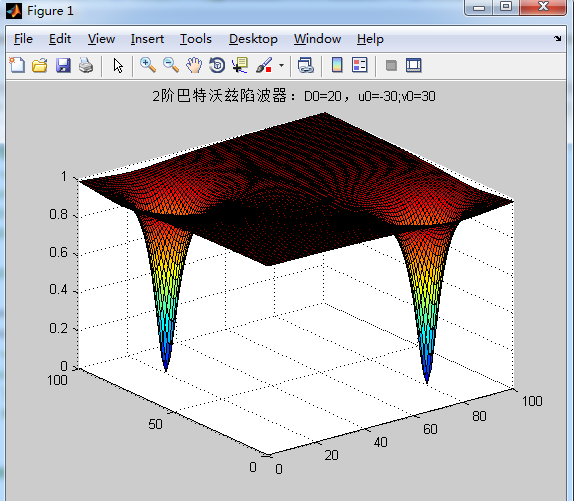代码：

[cpp] view
plain copya=100;%图像的尺寸，长
b=100;%图像的尺寸，宽
U=0:a;
V=0:b;
M=length(U);N=length(V);
D0=20;%W=200;%D0是频带的中心半径;W是频带的宽度
x1=10;y1=10;
u0=-30;v0=30;%（u0,v0）的值对应移动中心
m=fix(M/2);n=fix(N/2);
H=zeros(M,N);
n1=2;%巴特沃兹的阶数
W=10;%W是频带的宽度
for u=1:M
for v=1:N
D1=((U(u)-m-u0)^2+(V(v)-n-v0)^2)^0.5;
D2=((U(u)-m+u0)^2+(V(v)-n+v0)^2)^0.5;
temp=1+(D0^2/(D1*D2))^n1;
H(u,v)=1/temp;
end
end
figure;
surf(U,V,H)
title('2阶巴特沃兹陷波器：D0=20，u0=-30;v0=30')

3）高斯陷波器

传递函数：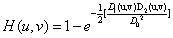透视图：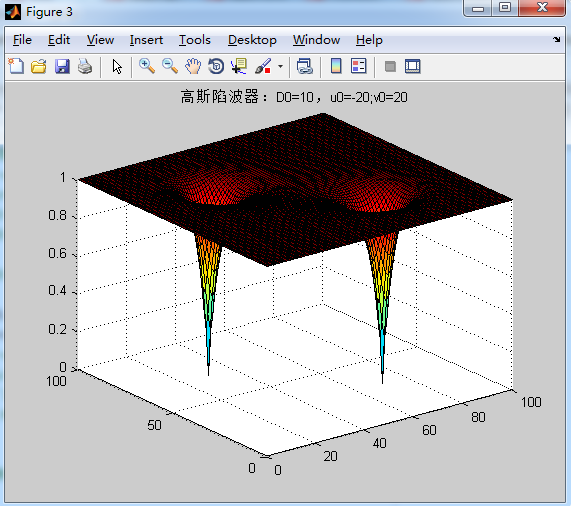代码：

[cpp] view
plain copy% %绘制函数剖面线
% [u,v] = meshgrid(-400:400, -400:400);
a=100;%图像的尺寸，长
b=100;%图像的尺寸，宽
U=0:a;
V=0:b;
M=length(U);N=length(V);
D0=10;%W=200;%D0是频带的中心半径;W是频带的宽度
x1=10;y1=10;
u0=-20;v0=20;%（u0,v0）的值对应移动中心
m=fix(M/2);n=fix(N/2);
H=zeros(M,N);
n1=2;%巴特沃兹的阶数
W=10;%W是频带的宽度
for u=1:M
for v=1:N
%         D1=((u-m-u0)^2+(v-n-v0).^2)^0.5;%（）
%         D2=((u-m+u0)^2+(v-n+v0).^2)^0.5;

D1=((U(u)-m-u0)^2+(V(v)-n-v0)^2)^0.5;%（）
D2=((U(u)-m+u0)^2+(V(v)-n+v0)^2)^0.5;
%         D11=((u-m-x1)^2+(v-n-y1).^2)^0.5;
%         D21=((u-m+x1)^2+(v-n+y1).^2)^0.5;

%         % %设计带阻滤波器
%         H(u,v)=1-exp(-0.5*((D(u,v)^2-D0^2)/(D(u,v)*W))^2);
%             D=sqrt((U(u) - m) .* (U(u)-m) + (V(v) - n) .* (V(v) - n));%D(u,v)的值
%             if((D<D0-W/2) || (D>D0+W/2))%理想带阻滤波器
%                 H(u,v)=1;
%             else
%                 H(u,v)=0;
%             end
temp=-0.5*(D1*D2/D0^2);
H(u,v)=1-exp(temp);
%         H(u,v)=1-exp(-0.5*((D1*D2/D0^2)));%尝试下，非理想滤波器，如巴特沃兹或者高斯
%       a=sqrt((U(u) - 50) .* (U(u)-50) + (V(v) - 50) .* (V(v) - 50));%D(u,v)的值
%       b=1+(a/D0)^2*n;
%       H(u,v)=1/b;%尝试下，非理想滤波器，如巴特沃兹或者高斯
end
end
%在绘制高斯曲面的时候，加上下述代码，显示得美观
% fangcha=50;
% H = -H/(2*fangcha);
% H = exp(H) / (sqrt(2*pi) * sqrt(fangcha));
figure;
surf(U,V,H)
title('高斯陷波器：D0=10，u0=-20;v0=20')
% [X,Y] = meshgrid(-2:.2:2, -2:.2:5);
% Z = X .* exp(-X.^2 - Y.^2);
% surf(X,Y,Z)


展开全文• 双二次或状态变量椭圆函数带通滤波节很适合于实现带阻传递函数，电路如图1所示。把电阻R，接到节点1或节点2，陷波频率凡将高于或低于极点频率（谐振频率）盂。 　所得到的节Q值可达200以上。设计参数fr、Q、f∞以及...
• 带阻滤波器传递函数为： D0为截止半径（或是截止宽度） D1、D2由下式确定，表示与（u0,v0）和（-u0,v0)点的距离，此时（u0,v0)是在频域中心为坐标原点表示的，所以是对称关系。 使用二阶巴斯沃特带阻滤波器对...
带阻滤波器的传递函数为：D0为截止半径（或是截止宽度）
D1、D2由下式确定，表示与（u0,v0）和（-u0,v0)点的距离，此时（u0,v0)是在频域中心为坐标原点表示的，所以是对称关系。使用二阶巴斯沃特带阻滤波器对周期噪声图像滤波：
噪声图像如下：首先观察其频谱，了解周期噪声分布：噪声分布在频域图像的竖直方向，在水平方向上没有。画频域图像中心竖直方向的频谱，得到噪声起始两点的位置，分别距离图像上边137和201，即u0=137,u1=201,v0=v,v1=v 。由此构造如下带阻滤波器滤波器和频域图像相乘得到以下图像最终得到的图像具体Matlab代码如下（以图像左上方为坐标原点）：
clc;
clear;
close all;

img=rgb2gray(img);
subplot(331),imshow(img),title('原图');
F=fftshift(fft2(img));
subplot(332),imshow(log(abs(F)+1),[]),title('频域图像');
[M,N]=size(F);
subplot(333),plot(abs(F(:,round(N/2)))),title('中心竖直线上频谱');
u=0:M-1;v=0:N-1;
[V,U]=meshgrid(v,u);
D0=14;
n=2;
u0=137;u1=201;
D1=abs(U-u0);
D2=abs(U-u1);
H=1./(1+(D0^2./(D1.*D2)).^n);
subplot(334),imshow(abs(H),[]),title('滤波器图像表示');
subplot(335),mesh(H),title('滤波器透视图');
G=F.*H;
subplot(336),imshow(log(abs(G)+1),[]),title('滤波后的频域图像');
g=real(ifft2(ifftshift(G)));
subplot(337),imshow(g,[]),title('滤波图像');



展开全文matlab
• 文章目录Python实现数字滤波器1、IIR低通、高通、带通和带阻滤波器的设计1.1、设计滤波器的函数1.2、将滤波器应用于语音 由语音的产生和感知可知，基音频率的范围是60到450赫兹之间，故语音信号采集需要提取基音时，...
Python实现数字滤波器
文章目录Python实现数字滤波器1、IIR低通、高通、带通和带阻滤波器的设计1.1、设计滤波器的函数1.2、将滤波器应用于语音
由语音的产生和感知可知，基音频率的范围是60到450赫兹之间，故语音信号采集需要提取基音时，需要采用低通滤波器来获取低频基音信号，在采用计算机采集语音信号时，语音常混有50赫兹交流混音，也需要采用高通滤波器将其去除，此篇设计数字滤波器，以及实现他们在语言中的简单应用。滤波器传递函数如下：
给定数字滤波器的M阶分子b和N阶分母a：
            jw                 -jw              -jwM
jw    B(e  )    b + be    + ... + b[M]e
H(e  ) = ------ = -----------------------------------
jw                 -jw              -jwN
A(e  )    a + ae    + ... + a[N]e

给定模拟滤波器的M阶分子b和N阶分母a：
        b*(jw)**M + b*(jw)**(M-1) + ... + b[M]
H(w) = ----------------------------------------------
a*(jw)**N + a*(jw)**(N-1) + ... + a[N]

1、IIR低通、高通、带通和带阻滤波器的设计
1.1、设计滤波器的函数
在python的scipy.signal库中给出了很多数字信号处理的函数参考：https://docs.scipy.org/doc/scipy/reference/signal.html#module-scipy.signal 可采用以下函数来设计数字滤波器。
（1）巴特沃斯滤波器
巴特沃斯数字和模拟滤波器设计函数：
butter(N, Wn[, btype, analog, output, fs])
功能：设计一个N阶数字或模拟巴特沃斯滤波器，然后返回滤波器系数。
调用格式：

b,a=scipy.signal.butter(N, Wn, btype=‘low’, analog=False, output=‘ba’, fs=None)
z,p,k=scipy.signal.butter(N, Wn, btype=‘low’, analog=False, output=‘zpk’, fs=None)
sos=scipy.signal.butter(N, Wn, btype=‘low’, analog=False, output=‘sos’, fs=None)

参数：

N（int）（滤波器阶次）
btype：（‘lowpass’,  ‘highpass’,  ‘bandpass’,  ‘bandstop’optional）（滤波类型，默认值是低通其中低通、高通、带通、带阻分别对应‘lowpass’,  ‘highpass’,  ‘bandpass’,  ‘bandstop’）
analog：（bool, optional）（为True时返回模拟滤波器，False时返回数字滤波器）
output：（‘ba’, ‘zpk’, ‘sos’optional）（向后兼容的输出类型）
fs：（float）（数字系统采用频率）

返回值：

b（ndarray）（传递函数分子）
a（ndarray）（传递函数分母）
z（ndarray）（零点）
p（ndarray）（极点）
k（float）（系统增益）
sos（ndarray）（IIR滤波器二阶部分表示）
巴特沃斯滤波器在通带中具有最大平坦的频率响应，如果要求传递函数形式，则由于根与多项式系数之间的转换是数值敏感的运算，即使对于N> = 4，也会出现数值问题。建议使用SOS表示形式。

绘制模拟滤波器频率响应：
计算频率响应的语句：
w, h = signal.freqs(b, a)#计算模拟滤波器的频率响应，返回h为频率响应，w为h的角频率
w, h = signal.freqz(b, a, worN=512, whole=False, plot=None, fs=6.283185307179586, include_nyquist=False)#计算数字滤波器的频率响应，返回h为频率响应（复数表示）w为h的角频率单位与fs相同默认情况下，w归一化为范围[0，pi）（弧度/样本），include_nyquist包含最后一个频率（奈奎斯特频率）
filtered = signal.sosfilt(sos, sig)#使用级联的二阶节沿一维过滤数据

import numpy as np
from scipy import signal
import matplotlib.pyplot as plt
b, a = signal.butter(4, 100, 'low', analog=True)  # 4阶低通临界频率为100Hz
w, h = signal.freqs(b, a)  # h为频率响应,w为频率
plt.figure(1)
plt.semilogx(w, 20 * np.log10(abs(h)))  # 用于绘制折线图，两个函数的 x 轴、y 轴分别是指数型的，并且转化为分贝
plt.title('Butterworth filter frequency response')
plt.ylabel('Amplitude [dB]')
plt.margins(0, 0.1)  # 去除画布四周白边
plt.grid(which='both', axis='both')  # 生成网格，matplotlin.pyplot.grid(b, which, axis, color, linestyle, linewidth， **kwargs)， which : 取值为'major', 'minor'， 'both'
plt.axvline(100, color='green')  # 绘制竖线
plt.show()
t = np.linspace(0, 1, 1000, False)  # 1秒，1000赫兹刻度
sig = np.sin(2*np.pi*10*t) + np.sin(2*np.pi*20*t)  # 合成信号
fig, (ax1, ax2) = plt.subplots(2, 1, sharex=True)  # 2行1列的图
ax1.plot(t, sig)
ax1.set_title('10 Hz and 20 Hz sinusoids')
ax1.axis([0, 1, -2, 2])  # 坐标范围生成由10 Hz和20 Hz组成的信号，以1 kHz采样
t = np.linspace(0, 1, 1000, False)  # 1秒，1000赫兹刻度
sig = np.sin(2*np.pi*10*t) + np.sin(2*np.pi*20*t)  # 合成信号
fig, (ax1, ax2) = plt.subplots(2, 1, sharex=True)  # 2行1列的图
ax1.plot(t, sig)
ax1.set_title('10 Hz and 20 Hz sinusoids')
ax1.axis([0, 1, -2, 2])  # 坐标范围

设计一个15 Hz的数字高通滤波器以消除10 Hz的音调，并将其应用于信号。（建议在过滤时使用二阶节格式，以避免传递函数（ba）格式出现数值错误）：
#sos = signal.butter(10, 15, 'hp', fs=1000, output='sos')  #10阶，15赫兹
sos = signal.butter(10, 15, btype='low', analog=False, output='sos', fs=1000)
filtered = signal.sosfilt(sos, sig)  # 滤波
ax2.plot(t, filtered)
ax2.set_title('After 15 Hz high-pass filter')
ax2.axis([0, 1, -2, 2])
ax2.set_xlabel('Time [seconds]')
plt.tight_layout()
plt.show()巴特沃斯滤波器阶次选择函数：
buttord(wp, ws, gpass, gstop[, analog, fs])
功能：返回最低阶数字或模拟巴特沃思滤波器的阶次，该滤波器在通带中的损失不超过gpass dB，在阻带中具有至少 gstop dB的衰减。
调用格式：

N, Wn = signal.buttord(wp, ws, gpass, gstop, analog=False, fs=None)

参数：

Wp（float）（通带边缘频率）
Ws（float）（阻带边缘频率）
对于数字滤波器，它们的单位与fs相同。默认情况下， fs是2个半周期/样本，因此将它们从0标准化为1，其中1是奈奎斯特频率。（因此，wp和ws在半周期/样本中。）例如：

低通：wp = 0.2，ws = 0.3
高通：wp = 0.3，ws = 0.2
带通：wp = [0.2，0.5]，ws = [0.1，0.6]
带阻：wp = [0.1，0.6]，ws = [0.2，0.5]

gpass（float）（通带中的最大损耗dB）

gstop（float）（阻带中的最小衰减dB）

analog：（bool, optional）（为True时返回模拟滤波器，False时返回数字滤波器）

output：（‘ba’, ‘zpk’, ‘sos’optional）（向后兼容的输出类型）

fs：（float）（数字系统采用频率）

返回值：

N（int）（滤波器阶次）

import numpy as np
from scipy import signal
import matplotlib.pyplot as plt
N, Wn = signal.buttord([20, 50], [14, 60], 3, 40, True)
b, a = signal.butter(N, Wn, 'band', True)
w, h = signal.freqs(b, a, np.logspace(1, 2, 500))
plt.semilogx(w, 20 * np.log10(abs(h)))  # 用于绘制折线图，两个函数的 x 轴、y 轴分别是指数型的，并且转化为分贝
plt.title('Butterworth bandpass filter fit to constraints')
plt.ylabel('Amplitude [dB]')
plt.grid(which='both', axis='both')  # 显示网格
plt.fill([1,  14,  14,   1], [-40, -40, 99, 99], '0.9', lw=0)  # 区域颜色填充阻带
plt.fill([20, 20,  50,  50], [-99, -3, -3, -99], facecolor='gray', alpha=0.2) # 通带，[x_左下, x_左上,  x_右上, x_右下(左下角顺时针)], [y_左下, y_左上,  y_右上, y_右下]
plt.fill([60, 60, 1e9, 1e9], [99, -40, -40, 99], '0.9', lw=0)  # 阻带
plt.axis([10, 100, -60, 3])  # 画布大小
plt.show()（2）切比雪夫I型滤波器
切比雪夫I型数字和模拟滤波器设计函数：
cheby1(N, rp, Wn[, btype, analog, output, fs])
功能：设计一个N阶数字或模拟切比雪夫I型滤波器，然后返回滤波器系数。
调用格式：

b,a=scipy.signal.cheby1(N, rp, Wn, btype=‘low’, analog=False, output=‘ba’, fs=None)
z,p,k=scipy.signal.cheby1(N, rp, Wn, btype=‘low’, analog=False, output=‘zpa’, fs=None)
sos=scipy.signal.cheby1(N, rp, Wn, btype=‘low’, analog=False, output=‘sos’, fs=None)

参数：

N（int）（滤波器阶次）
rp（float）（通带内允许的最大纹波低于单位增益。以分贝指定，为正数。）
btype：（‘lowpass’,  ‘highpass’,  ‘bandpass’,  ‘bandstop’optional）（滤波类型，默认值是低通其中低通、高通、带通、带阻分别对应‘lowpass’,  ‘highpass’,  ‘bandpass’,  ‘bandstop’默认值为低通）
analog：（bool, optional）（为True时返回模拟滤波器，False时返回数字滤波器）
output：（‘ba’, ‘zpk’, ‘sos’optional）（向后兼容的输出类型）
fs：（float）（数字系统采用频率）

返回值：

b（ndarray）（传递函数分子）
a（ndarray）（传递函数分母）
z（ndarray）（零点）
p（ndarray）（极点）
k（float）（系统增益）
sos（ndarray）（IIR滤波器二阶部分表示）

Chebyshev I型滤波器最大程度地提高了频率响应的通带和阻带之间的截止速率，但代价是通带中的纹波和阶跃响应中的振铃增加；I型滤波器比II型（cheby2）滚降的速度更快，但II型滤波器的通带中没有任何波动；等纹波通带具有N个最大值或最小值。
设计一个模拟滤波器并绘制其频率响应，显示关键点：
import numpy as np
from scipy import signal
import matplotlib.pyplot as plt
b, a = signal.cheby1(4, 5, 100, 'low', analog=True)
w, h = signal.freqs(b, a)
plt.semilogx(w, 20 * np.log10(abs(h)))
plt.title('Chebyshev Type I frequency response (rp=5)')
plt.ylabel('Amplitude [dB]')
plt.margins(0, 0.1)  # 去除画布四周白边
plt.grid(which='both', axis='both')
plt.axvline(100, color='green')  # # 绘制竖线，低通临界频率为100Hz
plt.axhline(-5, color='green')  # 绘制横线-rp
plt.show()生成由10 Hz和20 Hz组成的信号，以1 kHz采样
t = np.linspace(0, 1, 1000, False)
sig = np.sin(2*np.pi*10*t) + np.sin(2*np.pi*20*t)
fig, (ax1, ax2) = plt.subplots(2, 1, sharex=True)
ax1.plot(t, sig)
ax1.set_title('10 Hz and 20 Hz sinusoids')
ax1.axis([0, 1, -2, 2])

设计一个15 Hz的数字高通滤波器以消除10 Hz的音调，并将其应用于信号。（建议在过滤时使用二阶节格式，以避免传递函数（ba）格式出现数值错误）：
sos = signal.cheby1(10, 1, 15, 'hp', fs=1000, output='sos')
filtered = signal.sosfilt(sos, sig)
ax2.plot(t, filtered)
ax2.set_title('After 15 Hz high-pass filter')
ax2.axis([0, 1, -2, 2])
ax2.set_xlabel('Time [seconds]')
plt.tight_layout()
plt.show()切比雪夫I型滤波器阶次选择函数：
cheb1ord(wp, ws, gpass, gstop[, analog, fs])
调用格式：

N, Wn = scipy.signal.cheb1ord(wp, ws, gpass, gstop, analog=False, fs=None)

参数：

Wp（float）（通带边缘频率）
Ws（float）（阻带边缘频率）
对于数字滤波器，它们的单位与fs相同。默认情况下， fs是2个半周期/样本，因此将它们从0标准化为1，其中1是奈奎斯特频率。（因此，wp和ws在半周期/样本中。）例如：

低通：wp = 0.2，ws = 0.3
高通：wp = 0.3，ws = 0.2
带通：wp = [0.2，0.5]，ws = [0.1，0.6]
带阻：wp = [0.1，0.6]，ws = [0.2，0.5]

gpass（float）（通带中的最大损耗dB）

gstop（float）（阻带中的最小衰减dB）

analog：（bool, optional）（为True时返回模拟滤波器，False时返回数字滤波器）

output：（‘ba’, ‘zpk’, ‘sos’optional）（向后兼容的输出类型）

fs：（float）（数字系统采用频率）

返回值：

N（int）（滤波器阶次）
Wn（array、int）（切比雪夫固有频率（“ 3dB频率”）用于 cheby1给出滤波器结果。如果指定了fs，则其单位相同，并且还必须将fs传递给cheby1）

设计一个数字低通滤波器，使通带在3 dB以内，高达0.2 *（fs / 2），而在至少0.3dB（fs / 2）以上的频率下，至少要抑制-40 dB。绘制其频率响应，以灰色显示通带和阻带约束。
import numpy as np
from scipy import signal
import matplotlib.pyplot as plt
N, Wn = signal.cheb1ord(0.2, 0.3, 3, 40)
b, a = signal.cheby1(N, 3, Wn, 'low')
w, h = signal.freqz(b, a)
plt.semilogx(w / np.pi, 20 * np.log10(abs(h)))
plt.title('Chebyshev I lowpass filter fit to constraints')
plt.xlabel('Normalized frequency')
plt.ylabel('Amplitude [dB]')
plt.grid(which='both', axis='both')
plt.fill([.01, 0.2, 0.2, .01], [-3, -3, -99, -99], facecolor='gray', alpha=0.2) # 通带，[x_左下, x_左上,  x_右上, x_右下(左下角顺时针)], [y_左下, y_左上,  y_右上, y_右下]
plt.fill([0.3, 0.3,   2,   2], [ 9, -40, -40,  9], '0.9', lw=0)
plt.axis([0.08, 1, -60, 3])
plt.show()（3）切比雪夫II型滤波器
切比雪夫II型数字和模拟滤波器设计函数：
cheby2(N, rs, Wn[, btype, analog, output, fs])
功能：设计一个N阶数字或模拟切比雪夫II型滤波器，然后返回滤波器系数。
调用格式：

b,a=scipy.signal.cheby2(N, rs, Wn, btype=‘low’, analog=False, output=‘ba’, fs=None)
z,p,k=scipy.signal.cheby2(N, rs, Wn, btype=‘low’, analog=False, output=‘zpa’, fs=None)
sos=scipy.signal.cheby2(N, rs, Wn, btype=‘low’, analog=False, output=‘sos’, fs=None)

参数：

N（int）（滤波器阶次）
rs（float）（阻带所需的最小衰减。以分贝指定，为正数。）
btype：（‘lowpass’,  ‘highpass’,  ‘bandpass’,  ‘bandstop’optional）（滤波类型，默认值是低通其中低通、高通、带通、带阻分别对应‘lowpass’,  ‘highpass’,  ‘bandpass’,  ‘bandstop’默认值为低通）
analog：（bool, optional）（为True时返回模拟滤波器，False时返回数字滤波器）
output：（‘ba’, ‘zpk’, ‘sos’optional）（向后兼容的输出类型）
fs：（float）（数字系统采用频率）

返回值：

b（ndarray）（传递函数分子）
a（ndarray）（传递函数分母）
z（ndarray）（零点）
p（ndarray）（极点）
k（float）（系统增益）
sos（ndarray）（IIR滤波器二阶部分表示）

Chebyshev II型滤波器最大程度地提高了频率响应的通带和阻带之间的截止速率，但以阻带中的纹波和阶跃响应中的振铃为代价，II型滤波器的滚降速度不如I（cheby1）
设计一个模拟滤波器并绘制其频率响应，显示关键点：
import numpy as np
from scipy import signal
import matplotlib.pyplot as plt
b, a = signal.cheby2(4, 40, 100, 'low', analog=True)
w, h = signal.freqs(b, a)
plt.semilogx(w, 20 * np.log10(abs(h)))
plt.title('Chebyshev Type II frequency response (rs=40)')
plt.ylabel('Amplitude [dB]')
plt.margins(0, 0.1)
plt.grid(which='both', axis='both')
plt.axvline(100, color='green')  # cutoff frequency
plt.axhline(-40, color='green')  # -rs
plt.show()设计一个17 Hz的数字高通滤波器，以消除10 Hz的音调，并将其应用于信号。（建议在过滤时使用二阶节格式，以避免传递函数（ba）格式出现数值错误）：
t = np.linspace(0, 1, 1000, False)
sig = np.sin(2*np.pi*10*t) + np.sin(2*np.pi*20*t)
fig, (ax1, ax2) = plt.subplots(2, 1, sharex=True)
ax1.plot(t, sig)
ax1.set_title('10 Hz and 20 Hz sinusoids')
ax1.axis([0, 1, -2, 2])


sos = signal.cheby2(12, 20, 17, 'hp', fs=1000, output='sos')
filtered = signal.sosfilt(sos, sig)
ax2.plot(t, filtered)
ax2.set_title('After 17 Hz high-pass filter')
ax2.axis([0, 1, -2, 2])
ax2.set_xlabel('Time [seconds]')
plt.show()切比雪夫II型滤波器阶次选择函数：
cheb2ord(wp, ws, gpass, gstop[, analog, fs])
调用格式：

N, Wn = scipy.signal.cheb2ord(wp, ws, gpass, gstop, analog=False, fs=None)

功能：
返回最低阶数字或模拟Chebyshev Type II滤波器的阶次，该滤波器在通带中损耗不超过gpass dB，在阻带中至少具有 gstop dB衰减。
参数：

与切比雪夫I大致一致

返回值：

与切比雪夫I大致一致

设计一个数字带阻滤波器，该滤波器能抑制从0.2 *（fs / 2）到0.5 *（fs / 2）的-60 dB，同时保持在0.1 *（fs / 2）以下或0.6 *（fs / 2）以下的3 dB之内。绘制其频率响应，以灰色显示通带和阻带约束。
import numpy as np
from scipy import signal
import matplotlib.pyplot as plt
N, Wn = signal.cheb2ord([0.1, 0.6], [0.2, 0.5], 3, 60)
b, a = signal.cheby2(N, 60, Wn, 'stop')
w, h = signal.freqz(b, a)
plt.semilogx(w / np.pi, 20 * np.log10(abs(h)))
plt.title('Chebyshev II bandstop filter fit to constraints')
plt.xlabel('Normalized frequency')
plt.ylabel('Amplitude [dB]')
plt.grid(which='both', axis='both')
plt.fill([.01, .1, .1, .01], [-3,  -3, -99, -99], '0.9', lw=0)
plt.fill([.2,  .2, .5,  .5], [ 9, -60, -60,   9], '0.9', lw=0)
plt.fill([.6,  .6,  2,   2], [-99, -3,  -3, -99], '0.9', lw=0)
plt.axis([0.06, 1, -80, 3])
plt.show()（4）椭圆滤波器
椭圆数字和模拟滤波器设计函数：
ellip(N, rp, rs, Wn[, btype, analog, output, fs])
功能：设计一个N阶数字或模拟切比雪夫II型滤波器，然后返回滤波器系数。
调用格式：

b,a=scipy.signal.ellip(N, rp, rs, Wn, btype=‘low’, analog=False, output=‘ba’, fs=None)
z,p,k=scipy.signal.ellip(N, rp, rs, Wn, btype=‘low’, analog=False, output=‘zpk’, fs=None)
sos=scipy.signal.ellip(N, rp, rs, Wn, btype=‘low’, analog=False, output=‘sos’, fs=None)

参数：

N（int）（滤波器阶次）

rp（int）（通带内允许的最大纹波低于单位增益。以分贝指定，为正数。）

rs（float）（阻带所需的最小衰减。以分贝指定，为正数。）

Wn（array、int）（临界频率对于低通和高通Wn是标量，对于带通和带阻Wn是长度为2的序列，对于椭圆滤波器，这是过渡带中的增益首先下降到*-rp以下的点*，对于数字滤波器，Wn与fs的单位相同。默认情况下， fs是2个半周期/样本，因此将它们从0标准化为1，其中1是奈奎斯特频率。（因此，Wn为半周期/样本。）

btype：（‘lowpass’,  ‘highpass’,  ‘bandpass’,  ‘bandstop’optional）（滤波类型，默认值是低通其中低通、高通、带通、带阻分别对应‘lowpass’,  ‘highpass’,  ‘bandpass’,  ‘bandstop’默认值为低通）

analog：（bool, optional）（为True时返回模拟滤波器，False时返回数字滤波器）

output：（‘ba’, ‘zpk’, ‘sos’optional）（向后兼容的输出类型）

fs：（float）（数字系统采用频率）

返回值：

b（ndarray）（传递函数分子）
a（ndarray）（传递函数分母）
z（ndarray）（零点）
p（ndarray）（极点）
k（float）（系统增益）
sos（ndarray）（IIR滤波器二阶部分表示）

椭圆滤波器也称为Cauer或Zolotarev滤波器，它以频率波动的通带和阻带之间的跃迁速率最大化，但二者均以脉动为代价，并且阶跃响应中的振铃增加；当rp接近0时，椭圆滤波器变成Chebyshev II型滤波器（cheby2）；当rs接近0时，它成为Chebyshev I型滤波器（cheby1）；当两者都接近0时，它就变成了Butterworth过滤器（butter）；等纹波通带具有N个最大值或最小值（例如，一个5阶滤波器具有3个最大值和2个最小值）；因此，对于奇数阶滤波器，DC增益为单位；对于偶数阶滤波器，DC增益为-rp dB。
设计一个模拟滤波器并绘制其频率响应，显示关键点：
import numpy as np
from scipy import signal
import matplotlib.pyplot as plt>>> b, a = signal.ellip(4, 5, 40, 100, 'low', analog=True)
w, h = signal.freqs(b, a)
plt.semilogx(w, 20 * np.log10(abs(h)))
plt.title('Elliptic filter frequency response (rp=5, rs=40)')
plt.ylabel('Amplitude [dB]')
plt.margins(0, 0.1)
plt.grid(which='both', axis='both')
plt.axvline(100, color='green') # cutoff frequency
plt.axhline(-40, color='green') # rs
plt.axhline(-5, color='green') # rp
plt.show()生成由10 Hz和20 Hz组成的信号，以1 kHz采样
t = np.linspace(0, 1, 1000, False)  # 1 second
sig = np.sin(2*np.pi*10*t) + np.sin(2*np.pi*20*t)
fig, (ax1, ax2) = plt.subplots(2, 1, sharex=True)
ax1.plot(t, sig)
ax1.set_title('10 Hz and 20 Hz sinusoids')
ax1.axis([0, 1, -2, 2])

设计一个17 Hz的数字高通滤波器，以消除10 Hz的音调，并将其应用于信号。（建议在过滤时使用二阶节格式，以避免传递函数（ba）格式出现数值错误）：
sos = signal.ellip(8, 1, 100, 17, 'hp', fs=1000, output='sos')
filtered = signal.sosfilt(sos, sig)
ax2.plot(t, filtered)
ax2.set_title('After 17 Hz high-pass filter')
ax2.axis([0, 1, -2, 2])
ax2.set_xlabel('Time [seconds]')
plt.tight_layout()
plt.show()椭圆滤波器阶次选择函数：
ellipord(wp, ws, gpass, gstop[, analog, fs])
功能：
返回最低阶数字或模拟椭圆滤波器的阶数，该阶数在通带中损失不超过gpass dB，在阻带中至少具有 gstop dB衰减。
调用格式：

N, Wn =scipy.signal.ellipord(wp, ws, gpass, gstop, analog=False, fs=None)

参数：

Wp（float）（通带边缘频率）
Ws（float）（阻带边缘频率）
对于数字滤波器，它们的单位与fs相同。默认情况下， fs是2个半周期/样本，因此将它们从0标准化为1，其中1是奈奎斯特频率。（因此，wp和ws在半周期/样本中。）例如：

低通：wp = 0.2，ws = 0.3
高通：wp = 0.3，ws = 0.2
带通：wp = [0.2，0.5]，ws = [0.1，0.6]
带阻：wp = [0.1，0.6]，ws = [0.2，0.5]

gpass（float）（通带中的最大损耗dB）

gstop（float）（阻带中的最小衰减dB）

analog：（bool, optional）（为True时返回模拟滤波器，False时返回数字滤波器）

output：（‘ba’, ‘zpk’, ‘sos’optional）（向后兼容的输出类型）

fs：（float）（数字系统采用频率）

返回值：

N（int）（滤波器阶次）
Wn（array、int）（切比雪夫固有频率（“ 3dB频率”）用于 ellip给出滤波器结果。如果指定了fs，则其单位相同，并且还必须将fs传递给ellip）

import numpy as np
from scipy import signal
import matplotlib.pyplot as plt
N, Wn = signal.ellipord(30, 10, 3, 60, True)
b, a = signal.ellip(N, 3, 60, Wn, 'high', True)
w, h = signal.freqs(b, a, np.logspace(0, 3, 500))
plt.semilogx(w, 20 * np.log10(abs(h)))
plt.title('Elliptical highpass filter fit to constraints')
plt.ylabel('Amplitude [dB]')
plt.grid(which='both', axis='both')
plt.fill([.1, 10,  10,  .1], [1e4, 1e4, -60, -60], '0.9', lw=0) # stop
plt.fill([30, 30, 1e9, 1e9], [-99,  -3,  -3, -99], '0.9', lw=0) # pass
plt.axis([1, 300, -80, 3])
plt.show()1.2、将滤波器应用于语音
设计一个切比雪夫II型滤波器，他的通带频率Wp为500Hz，阻带频率Ws为750Hz，采样频率为8000Hz，通带最大损耗Rp为3dB，阻带最小衰减为50dB,对bluesky31.wav数据进行滤波。
import numpy as np
import librosa, wave
from scipy import signal
import math
# 导入音频及绘图显示包
import librosa.display
# 导入绘图工作的函数集合
import matplotlib.pyplot as plt

times = librosa.get_duration(filename='./bluesky31.wav')  # 获取音频时长单位为秒
y0, sr = librosa.load('bluesky31.wav', sr=8000, offset=0.0, duration=None)  # 返回音频采样数组及采样率
print("sr=", sr)
print("times=", times)
# 绘图
PointNumbers = int(times * sr) + 1
x1 = np.arange(0, PointNumbers, 1)  # 采样点刻度
x2 = np.arange(0, times, 1 / sr)  # 时间刻度
plt.figure()
plt.xlabel("times")
plt.ylabel("amplitude")
plt.title('bluesky31.wav', fontsize=12, color='black')
plt.plot(x2, y0)
plt.show()

# 去除趋势化信息
def polydetrend(x, fs, m):
N = len(x)  # 信号长度
xtrend = np.zeros(len(x))  # 创建0数组
i = np.arange(0, N, 1)  # 时间刻度
k = i / fs  # 按信号长度取时间刻度
a = np.polyfit(k, x, m)  # 在此m为逼近多项式系数的次数，返回逼近后的信号序列多项式拟合系数值
xtrend[i] = np.polyval(a, k)  # 用系数构成趋势项
y = x - xtrend  # 从需要去趋势信息的信号中减去趋势项
return (y, xtrend)

y0, xtrend = polydetrend(y0, sr, 2)
plt.figure()
plt.xlabel("times")
plt.ylabel("amplitude")
plt.title('bluesky31.wav_Elimination_trend', fontsize=12, color='black')
plt.plot(x2, y0)
plt.show()
y1 = y0 - np.mean(y0)  # 消除直流分量
y1 = y1 / np.max(np.abs(y1))  # 幅值归一化
plt.figure()
plt.xlabel("times")
plt.ylabel("amplitude")
plt.title('The normalized bluesky31.wav', fontsize=12, color='black')
plt.plot(x2, y1)
plt.show()

sr= 8000
times= 2.375# 设计切比雪夫II型滤波器
fp = 500  # 滤波器通带频率
fs1 = 750  # 滤波器阻带频率
sr2 = sr/2  # 采样频率半周期每样本
Wp = fp/sr2  # 通带频率归一化
Ws = fs1/sr2  # 阻带频率归一化
Rp = 3  # 通带最大损耗
Rs = 50  # 阻带最小衰减
n, Wn = signal.cheb2ord(Wp, Ws, Rp, Rs, analog=False, fs=None)  #当参数未归一化时，fs=sr
b, a = signal.cheby2(n, Rs, Wn, btype='lowpass', analog=False, output='ba', fs=None)
sos = signal.cheby2(n, Rs, Wn, btype='lowpass', analog=False, output='sos', fs=None)
w, h = signal.freqz(b, a ,fs=sr)  # 返回的w单位与参数fs相同
plt.plot(w, 20 * np.log10(abs(h)))
plt.title('Chebyshev Type II frequency response')
plt.xlabel('Frequency [Hz]')
plt.ylabel('Amplitude [dB]')
plt.margins(0, 0.1)  # 去除画布四周白边
plt.grid(which='both', axis='both')#网格
plt.axvline(Wn*sr2, color='green')  # 绘制竖线，低通截止频率(取归一化)
plt.axhline(-Rs, color='green')  # 绘制横线，阻带衰减
plt.fill([fs1,  fs1,  sr2,   sr2], [-Rs, 20,  20, -Rs], '0.9', lw=0)  # 阻带约束
plt.fill([0,  0, fp, fp], [ -100, -Rp, -Rp,   -100], '0.9', lw=0)  # 通带约束
plt.show()# 对语音信号滤波
filtered = signal.sosfilt(sos, y1)
fig, (ax1, ax2) = plt.subplots(2, 1, sharex=True)
ax1.plot(x2, y1)
ax1.set_title('The normalized bluesky31.wav')
ax1.axis([0, times, -1, 1])
ax1.set_ylabel('Amplitude')
ax2.plot(x2, filtered)
ax2.set_title('After 500 Hz cheby2 low-pass filter')
ax2.axis([0, times, -1, 1])
ax2.set_xlabel('Time [seconds]')
ax2.set_ylabel('Amplitude')
plt.show()# 信号的语谱
LenFrame=200#每帧长
PatFrame=80#帧移
nfft=512
window = signal.windows.hann(LenFrame)
f1, t1, Zxx1 = signal.stft(y1, fs=sr,window=window,nperseg=LenFrame,noverlap=PatFrame,nfft=nfft)  #返回频率刻度序列（1D张量），时间刻度序列（1D张量），以及filtered的短时傅里叶变换（2D张量）
f2, t2, Zxx2 = signal.stft(filtered, fs=sr,window=window,nperseg=LenFrame,noverlap=80,nfft=nfft)

fig, (ax1, ax2) = plt.subplots(2, 1, sharex=True)
ax1.pcolormesh(t1, f1, np.abs(Zxx1))  #绘制背景图
ax1.set_title('The normalized bluesky31.wav of Language spectra')
ax1.set_ylabel('Frequncy [Hz]')

ax2.pcolormesh(t2, f2, np.abs(Zxx2))
ax2.set_title('After 500 Hz cheby2 low-pass filter of Language spectra')
ax2.set_xlabel('Time [seconds]')
ax2.set_ylabel('Frequncy [Hz]')
plt.show()展开全文python 信号处理
• 1.滤波器工程上根据输出端口对信号频率...分类分类：滤滤波器按滤波特性可分为低通、高通、带通和带阻四类，其传递函数如图所示。低通滤波器，指低频信号能通过而高频信号不能通过的滤波器；高通滤波器，指高频信号...
• 原标题：一次弄懂低通、高通、带通、带阻、状态可调滤波器!低通滤波器1 二阶压控低通滤波器二阶压控低通滤波器电路如图所示，由R1、C1 及R2、C2 分别构成两个一阶低通滤波器，但C1 接输出端，引入电压正反馈，形成压...
• （1）理想带阻理想带阻滤波器传递函数为： 其中C0是截止频率，W是带宽，即从截止频率两侧宽W/2的区域叫做阻止带。理想带阻可以看出理想带阻滤波器就是一个高通滤波器（图左侧）和一个低通滤波器（图中右侧曲线）组成...
• (1) 传递函数(2) 频率特性可见该低通滤波器特点是阻尼系数ζ 由电阻R1、R2，C1、C2 的比决定；而固有频率ω0与R1、R2、C1、C2 具体数值有关，即ω0与ζ 独立可调，互不影响。(3) 参数选择为方便参数匹配，考虑到标称...
• (1) 传递函数(2) 频率特性可见该低通滤波器特点是阻尼系数ζ 由电阻R1、R2，C1、C2 的比决定；而固有频率ω0与R1、R2、C1、C2 具体数值有关，即ω0与ζ 独立可调，互不影响。(3) 参数选择为方便参数匹配，考虑到标称...
• (1) 传递函数(2) 频率特性可见该低通滤波器特点是阻尼系数ζ 由电阻R1、R2，C1、C2 的比决定；而固有频率ω0与R1、R2、C1、C2 具体数值有关，即ω0与ζ 独立可调，互不影响。(3) 参数选择为方便参数匹配，考虑到标称...
• 　带阻滤波器的设计是首先把归一化低通滤波器变换成高通网络，其截止频率等于要求的带宽，并且有需要的阻抗。然后用同样的方法，把每个高通元仵调谐到中心频率上。 　这相当于一个新的变量代替高通传递函数中的频率...
• 如何弄懂低通、高通、带通、带阻、状态可调滤波器。【高通滤波器】-二阶高通滤波器传递函数的一般形式；二阶高通压控滤波器；通带增益Aup为1 的二阶压控高通滤波器；无限增益多路反馈高通滤波器；二阶高通滤波器。
• 1．频率归一化　在设计滤波器的传递...传递函数的幅度近似　在设计、研究滤波器时，通常是按通频带分类，分为低通滤波器、高通滤波器、带通滤波器、带阻滤波器。在这4种滤波器中，常将低通滤波器作为设计滤波器的基础
• 　(1) 传递函数 　(2) 频率特性 　可见该低通滤波器特点是阻尼系数ζ 由电阻R1、R2，C1、C2 的比决定；而固有频率ω0与R1、R2、C1、C2 具体数值有关，即ω0与ζ 独立可调，互不影响。...
• 1二阶压控低通滤波器 ...(1) 传递函数 (2) 频率特性 可见该低通滤波器特点是阻尼系数ζ 由电阻R1、R2，C1、C2 的比决定；而固有频率ω0与R1、R2、C1、C2 具体数值有关，即ω0与ζ 独立可调，互不影响。 ...
• (1) 传递函数(2) 频率特性可见该低通滤波器特点是阻尼系数ζ 由电阻R1、R2，C1、C2 的比决定；而固有频率ω0与R1、R2、C1、C2 具体数值有关，即ω0与ζ 独立可调，互不影响。(3) 参数选择为方便参数匹配，考虑到标称...
• (1) 传递函数(2) 频率特性可见该低通滤波器特点是阻尼系数ζ 由电阻R1、R2，C1、C2 的比决定；而固有频率ω0与R1、R2、C1、C2 具体数值有关，即ω0与ζ 独立可调，互不影响。(3) 参数选择为方便参数匹配，考虑到标称...
• 在设计有源滤波器时，一般遵从以下设计步骤。　1，传递函数的设计　根据对滤波器特性的要求，设计...若要求设计带阻滤波器时，再将低通原型传递函数变换为带阻目标传递函数。　2．电路设计　按各个低阶传递函数的设计
• 用组合低通和高通网络...　这个变换将低通到高通和其后的带阻变换结合在一起，使得带阻滤波器能直接由低通传递函数得到。 　每对低通复数极点在带阻变换时产生两对复数极点和一对2阶虚数零点。单个低通实数极点变换时
• 2．四种滤波器的传递函数和实验模拟电路如图4-2 所示：(a)无源低通滤波器 (b)有源低通滤波器(c) 无源高通滤波器 (d)有源高通滤波器(e)无源带通滤波器 (f)有源带通滤波器(g)无源带阻滤波器 (h)有源带阻滤波器 ...
• 上一篇文章1 讨论了滤波器相位与滤波器实现拓扑之间的关系，本篇将讨论滤波器传递函数本身的相位漂移。虽然滤波器主要是针对幅度响应而设计的，但在延时仿真、级联滤波器电路特别是过程控制环路等应用中相位响应非常...
• 按其幅频特性可分为低通、高通、带通和带阻滤波器四种电路。应用时应根据有用信号、无用信号和干扰等所占频段来选择合理的类型。2. 有源滤波电路一般均引入电压负反馈,因而集成运放工作在线性区,故分析方法与运算...
• 最终画出低通滤波器的幅频、相频特性如图1(a)所示。实际电路采用由Max291...在Matlab中用[b，a]=butter(n，Wn，′stop′，′s′)语句来实现带阻滤波器，其中[b，a]是滤波器传递函数多项式的系数；n为滤波器的阶数...